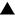Algebra 1 section of MathBitsNotebook.comRadicals (emphasis on square roots) Terms of Use | Contact Person: Donna Robertsindicates compatibility to NY Next Generation Math Standards (NGMS)
*
Remembering Radicals (general info, square/cube roots, estimating)*
Simplify Radicals - Numerical (primarily square roots)PRACTICE: • Simplifying Radicals (numerical square roots)• Add and Subtract Radicals (numerical square roots)• Multiply and Divide Radicals (numerical square roots, rationalize)• Arithmetic of Radicals (numerical square roots, +, -, x, ÷)• Working with Cube Roots (introductory work only) • Working with Negative Square Roots (introductory work only) • Vocabulary - Word Guess Game (topical vocabulary game - iPad compatible)